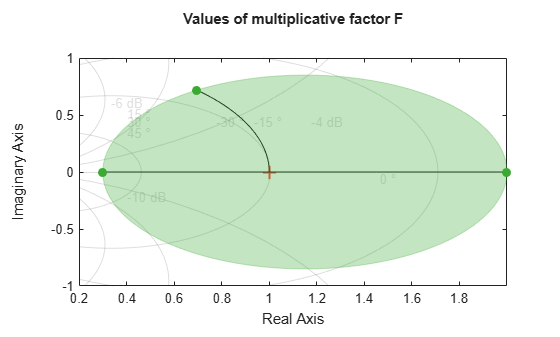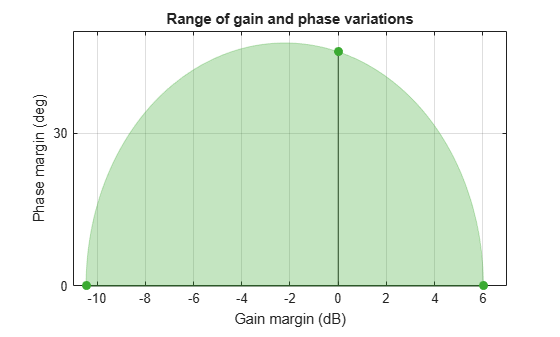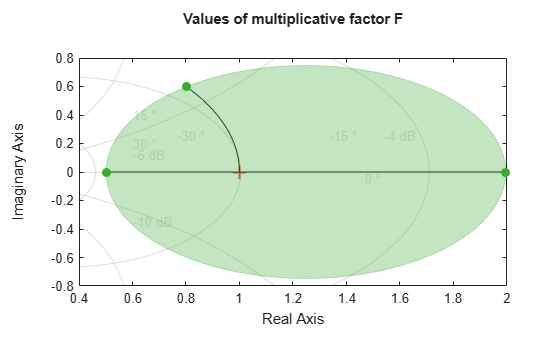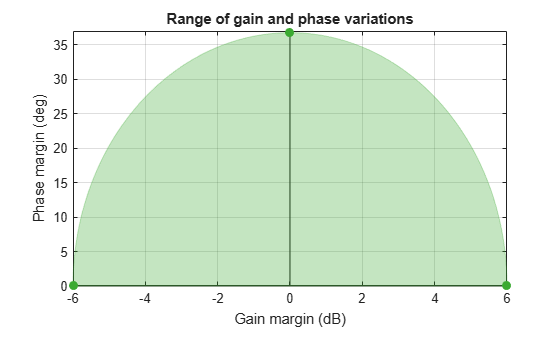getDPM

Disk-based phase variation corresponding to disk-based gain variation

Description

example

DPM = getDPM(DGM) returns the disk-based phase variation corresponding to disk-based gain variation DGM. In the model used by umargin, gain and phase variation are represented as a multiplicative factor F(s) taking values in a disk centered on the real axis. The disk described by its real-axis intercepts DGM = [gmin,gmax], which represent the relative amount of gain variation around the nominal value F = 1. Because the disk is complex-valued, the disk described by DGM also represents a certain amount of phase variation, DPM. For more information, see getDGM.

example

DPM = getDPM(GM) is the same as getDPM([1/GM,GM]). This syntax returns the disk-based phase variation range corresponding to a gain that can increase or decrease by a factor GM.

Examples

collapse all

Find the disk-based phase margin which corresponds to the disk-based gain margin with variations in the range [0.3,2].

DGM = [0.3,2];
DPM = getDPM(DGM)
DPM = 1×2

-45.9208   45.9208

DGM = [0.3,2] describes a disk of multiplicative gain and phase uncertainty in which the gain can vary from 0.3 times the nominal value to twice the nominal value (at nominal phase). Visualize the disk.

diskmarginplot(DGM,'disk')The disk also describes phase variations of about ±46°. getDPM returns this phase range as DPM, which is the range in which the modeled phase can vary at nominal gain. Visualize the range of simultaneous gain and phase variations captured in the disk described by DGM = [0.3,2] and DPM = [-45.92 45.92].

diskmarginplot(DGM)Find the disk-based phase margin corresponding to the gain variation of ±6 dB, or a factor of 2 in either direction.

GM = db2mag(6);
DPM = getDPM(GM)
DPM = 1×2

-36.7611   36.7611

For a scalar input GM, getDPM(GM) is same as getDPM([1/GM,GM]).

DPM = getDPM([1/GM,GM])
DPM = 1×2

-36.7611   36.7611

For the given gain variation of ±6 dB, the corresponding disk models the phase variation range of about ±36.8° at nominal gain. Visualize the disk and the combined gain and phase variations it represents.

diskmarginplot([1/GM,GM],'disk')diskmarginplot([1/GM,GM])Input Arguments

collapse all

Range of relative gain variation, specified as a two-element vector of the form [gmin,gmax], where gmin < 1 and gmax > 1. For instance, DGM = [0.8 1.5] represents a gain that can vary between 80% and 150% of its nominal value (that is, change by a factor between 0.8 and 1.5). gmin can be negative, defining a range of relative gain variation that includes a change in sign.

DGM represents that the disk intersects real axis in the interval [gmin,gmax], where gmin < 1 and gmax > 1. For instance, the following plot shows a disk characterized by DGM = [0.5,2].You can obtain DGM from desired gain and phase variations (or margins) using getDGM. The GainMargin field of the output structures of the diskmargin command is also a disk-based gain range of this form. .

To get DPM corresponding to multiple gain ranges at once, specify is a two-column matrix of form [gmin1,gmax1;...;gminN,gmaxN].

Amount of gain increase or decrease, specified as a real scalar or column vector.

• If GM is a real scalar, then getDPM returns the disk-based phase variation corresponding to a symmetric gain variation of [1/GM,GM]. For instance, GM = 2 specifies a gain that can increase or decrease by a factor of 2.

• If GM is a vector of form [GM1;...;GMN], then the function returns disk-based phase variations corresponding to each range [1/GM1,GM1;...;1/GMN,GMN].

Output Arguments

collapse all

Disk-based phase variation, returned as a two-element vector or a two-column matrix.

The vector DPM = [-pm,pm], represents the relative phase variation amount determined by the geometry of the disk described by DGM. For more information, see getDGM.

If DGM is a two-column matrix containing multiple gain-variation ranges, the function returns a two-column matrix of the form [-pm1,pm1; ...;-pmN,pmN].